# Weakly Supervised Learning for Object Localization

Supervised learning techniques require large quantities of high-quality annotations as labels. For tasks like segmentation, the time and cost of creating annotations are often higher than tasks like classification. To overcome these limitations, one low-cost option is to make use of lower quality annotations collected in large quantities, i.e using weakly supervised learning.

In this article, we’re going to focus on applying WSL techniques to image-based data, to be precise, WSL for object Localization.

# Object Localization

Object localization refers to identifying the location of one or more objects in an image and drawing a bounding box around their extent. …

# Weakly Supervised Learning: Overture

## Introduction

The domain of AI has grown drastically in recent years, generating state-of-the-art models. However, most of these models rely on massive sets of hand-labeled data. This hard-labor-work is extremely time-consuming and expensive: may require person-months or years to clean and assemble data. Not to forget, the data even evolves in the real-world and might need time-to-time updates.
For the above-mentioned reasons, practitioners are turning towards a weaker form of supervision: generating training data with different patterns, rules, external knowledge, or other classifiers. These all are the ways of generating the training data.

# Pruning in Deep Learning: The efficacy of pruning for model compression

Through this article, we will seek to perform a closer exploration of the effectiveness of pruning for model compression.Source

# Introduction

The primary property of deep learning is that its accuracy empirically scales with the size of the model and the amount of training data. This property has dramatically improved the state-of-the-art performance across sectors. With that, the resources required for the training and serving these models scales with model and data size. The equivalence of scale to model quality is the catalyst for the pursuit of efficiency in the machine learning and systems community. A promising avenue for improving the efficiency…

# k-means Clustering Algorithm: Explained and Implemented

This article will help you understand another unsupervised ML algorithm to solve clustering problems. Let’s begin.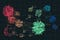Source

# Introduction

k-means is one of the mildest unsupervised learning algorithms used to solve the well-known clustering problem. It is an iterative algorithm that tries to partition the dataset into a ‘k’ number of pre-defined distinct non-overlapping subgroups (clusters). The main idea is to define k centers, one for each cluster. These centers should be placed in a crafty way because of different location causes the different result. So, the better choice is to place them as much as possible far away from each other. …

# Naive Bayes: Explained and Implemented

Through this article, we’re going to learn about the working of another traditional ML algorithm named ‘Naive Bayes’.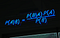Source.

# Introduction

The Naive Bayes algorithm is a classification technique based on Bayes’ Theorem with an assumption of independence among predictors. Bayes theorem states that:

# Support Vector Machine: Explained and Implemented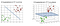2D and 3D Hyperplanes in SVM | Source

Through this article, you’ll learn about one of the widely used classifications and regression algorithms: Support Vector Machine (SVM). Assuming that the reader is already accustomed to Logistic regression. If not, you can refer to my article on LR.

# Introduction to SVM

An SVM model is another powerful yet flexible machine learning algorithm used for both classification and regression problems. It is primarily used for the classification objectives due to its great potential to handle multiple continuous and categorical variables.

# Introduction

Random Forests are an ensemble learning method that can be used for performing both regression and classification tasks. Random forests usually outperform decision trees, but their accuracy is lower than gradient boosted trees. It is also useful for dimensionality reduction methods, handling missing values, and outliers.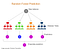Random Forest | Source

Decision trees are invariant under scaling and other transformations of feature values, hence robust to the inclusion of irrelevant features and can produce inspectable models.

To be precise, trees with great depth tend to learn a highly irregular pattern. …

# Logistic Regression — Explained and Implemented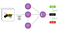Source

Through this article, we’re going to learn about Logistic Regression(LR). This article is divided into two parts:

1. Brief Explanation of Logistic Regression
2. Implementation of Logistic Regression

For those who have no idea regarding Logistic Regression, I’d suggest going through a more detailed article: Logistic Regression Explained

Let’s get started.

## 1. Brief Explanation of Logistic Regression

Despite having the word ‘regression in its name, Logistic Regression is a kind of binary classification algorithm. It is named ‘Logistic Regression’ because it’s similar to Linear Regression. The term “Logistic” is taken from the Logit function that is used in this method of classification.

It is a Binary-Classification technique, therefore…## Manmohan Dogra

AI Enthusiast | Independent researcher